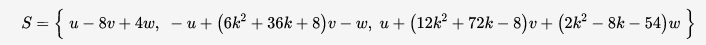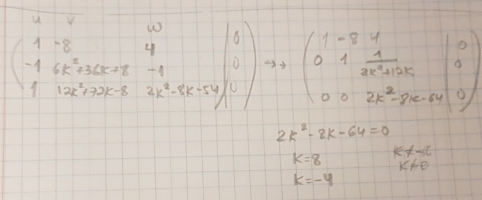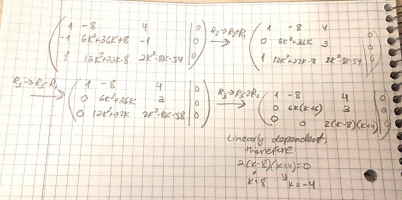# ?Did I Solve This Correctly

#### TheWrathOfMath

##### Junior Member
Given: u,v,w are linearly independent vectors.
Find all values of k for which the following set is linearly dependent:Is my solution correct?
I basically put all vectors in a matrix, changed it into (reduced) row echelon form using elementary row operations (I know that simply row echelon form is enough, but I decided to continue) and set the appropriate entry equal to zero so that R3 will be equal to zero.

I obtained only two values and I am not certain about whether I solved the problem properly.#### blamocur

##### Senior Member
Do you know how to verify your result? I.e., what do you get when you plug in the found values for [imath]k[/imath] into your expressions for vectors?

•Subhotosh Khan and Steven G

#### Steven G

##### Elite Member
Did you consider using determinants?

#### TheWrathOfMath

##### Junior Member
Did you consider using determinants?
We did not learn determinants yet, though I know what they are.

#### TheWrathOfMath

##### Junior Member
Do you know how to verify your result? I.e., what do you get when you plug in the found values for [imath]k[/imath] into your expressions for vectors?
These values work, but I wonder whether there is a possibility that I missed some values.

#### blamocur

##### Senior Member
I think you have missed some values. Why do you write [imath]k \neq 0[/imath] and [imath]k \neq - 6[/imath] ?

Your post is rather brief, so I can't tell how you got from one matrix to another. If you include intermediate steps then either you might discover on your own what is missing or we might be able to help you better.

•Steven G

#### TheWrathOfMath

##### Junior Member
I think you have missed some values. Why do you write [imath]k \neq 0[/imath] and [imath]k \neq - 6[/imath] ?

Your post is rather brief, so I can't tell how you got from one matrix to another. If you include intermediate steps then either you might discover on your own what is missing or we might be able to help you better.EDIT: I checked k=0 and the set is also linearly dependent for that value.
Perhaps I am missing additional values, though?

Last edited:

#### TheWrathOfMath

##### Junior Member
I think you have missed some values. Why do you write [imath]k \neq 0[/imath] and [imath]k \neq - 6[/imath] ?

Your post is rather brief, so I can't tell how you got from one matrix to another. If you include intermediate steps then either you might discover on your own what is missing or we might be able to help you better.
Never mind. I solved it using determinants, though I do not understand why my method is wrong.

#### blamocur

##### Senior Member

•Steven G

#### blamocur

##### Senior Member
I can see how you get to the point where it is easy to compute the determinant, but you said you are not supposed to use them -- what are you using? Can you explain your method or post a link to an explanation?

•Steven G

#### TheWrathOfMath

##### Junior Member
I can see how you get to the point where it is easy to compute the determinant, but you said you are not supposed to use them -- what are you using? Can you explain your method or post a link to an explanation?
Put the coefficients of the vectors in a matrix, change it to row echelon form and check for what values of k, at least one of the rows is all zero (since it means that the vectors are linearly dependent).

#### blamocur

##### Senior Member
Put the coefficients of the vectors in a matrix, change it to row echelon form and check for what values of k, at least one of the rows is all zero (since it means that the vectors are linearly dependent).
Having at least one of the rows all zero is too strong a condition, which explains why you are missing some of the answers. Once you get to a triangular matrix it enough to zero any of the diagonal elements (which is equivalent to have a 0 determinant).

•topsquark and Steven G